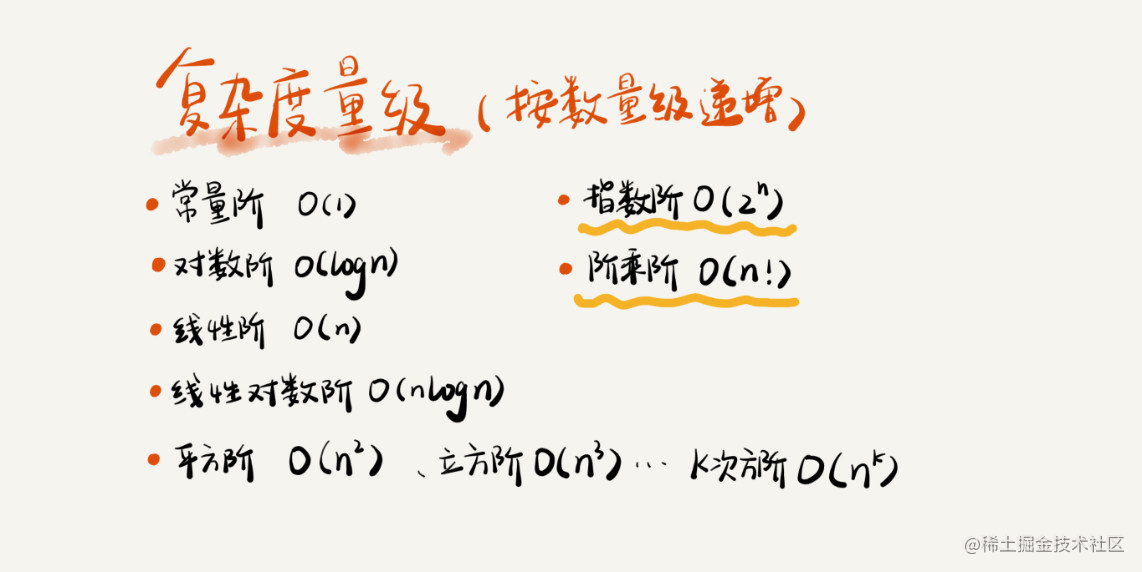# 结构结构&算法-时间空间复杂度分析笔记### 大O复杂度表示法

`````` int cal(int n) {
int sum = 0;
int i = 1;
for (; i <= n; ++i) {
sum = sum + i;
}
return sum;
}1.只关注循环执行次数最多的一段代码

`````` int cal(int n) {
int sum = 0;
int i = 1;
for (; i <= n; ++i) {
sum = sum + i;
}
return sum;
}

2.加法法则：总复杂度等于量级最大的那段代码的复杂度

3.乘法法则：嵌套代码的复杂度等于嵌套内外代码复杂度的乘积1. O(1)

2. O(logn)、O(nlogn)

`````` i=1;

while (i <= n)  {

i = i * 2;

}`````` i=1;
while (i <= n)  {
i = i * 3;
}